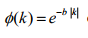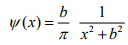# Error "Bad Numerical Expression k"

I want to draw these functions graphandI am using TF1 and I write this code

TF1 *tf1 = new TF1(“graph”,“exp(-k)”,0.0,0.5); for graph 1 but I have error which bad numerical expression k
How can I write code for this graph

Try

``````TF1 *tf1 = new TF1("graph","exp(-1.**TMath::Abs(x))",0.0,0.5);
``````

I tried but I think result is not true

Maybe your `` (the `b` parameter from your original example) is set to `0`? That would explain it. Try setting it to something different with `SetParameter()`.

setParameter Can you give example I am cnfused a bit

``````tf1->SetParameter(0, 10);
``````

will set the `` to `10`.

Thanks I think it is Ok this time

and I try my second graph now

TF1 ref guide explains all this very clearly:
https://root.cern/doc/master/classTF1.html

I tried my second graph like this

TF1 * f2 = new TF1(“graph” ,"(1.TMath::Abs(x)/90.0)(1/xx+b*TMath::Abs(x)b*TMath::Abs(x))",0.0,0.5);

but again I have numerical error ?

Why do you have `Abs(x)` this time? And why do you have `b` in the code?
`TF1* tf2 = new TF1("graph2", "/(TMath::Pi() * (x*x+*))", 0., 0.5)`

oov I am sorry Thank you

This topic was automatically closed 14 days after the last reply. New replies are no longer allowed.